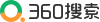# 发展求变，积极开拓海外市场

 随着国内经济下行趋势日益加剧，加之耐材行业自身存在的无序竞争、产能饱和等因素影响，去年国内耐火材料市场交易量也有比较明显的下滑，而由于我国耐火材料工业的技术进步和发展，我国的耐火材料产品品质得到了很大提高，具备技术创新能力的企业提供的大部分产品能够满足国际用户要求，国际企业出于自身利益的考虑，逐步加大向具有技术优势和成本优势的中国企业的采购规模。   在这机遇与(yu)挑战并(bing)存的背景下，我(wo)公司早在2014年就(jiu)已经开始(shi)着手布局国际市场(chang)。目(mu)前(qian)我(wo)公司已经陆续跟沙特、巴基斯坦、吉尔吉斯斯坦、越南、约旦、苏丹、也门等国家的(de)最终用户(hu)建立(li)了(le)合作(zuo)，并通过参加国际展会，与越来越多的(de)业务企业、专家建立(li)了(le)合作(zuo)关系(xi)。　　 “一味地靠低价(jia)厮(si)杀是没有(you)出(chu)路的(de)(de)，只有(you)在质量(liang)、服务(wu)和成本上提(ti)高内在实力，才能(neng)(neng)在国(guo)际市场(chang)的(de)(de)竞争中(zhong)夺得(de)(de)先(xian)机。”锦诚外贸部(bu)负责人王(wang)洪杰说。“针对(dui)中(zhong)东地区燃料使用的(de)(de)不同，我们及(ji)时对(dui)产品的(de)(de)化(hua)学成分(fen)及(ji)工艺参数进行了(le)(le)优化(hua)，对(dui)产品性能(neng)(neng)提(ti)出(chu)了(le)(le)新的(de)(de)要求，从(cong)而稳定了(le)(le)产品性能(neng)(neng)，只有(you)保证了(le)(le)产品质量(liang)，才可能(neng)(neng)赢得(de)(de)了(le)(le)客户(hu)。” 　　随着国家(jia)“一带一路”的(de)战略推行以(yi)及企业对国际(ji)市场的(de)理解日(ri)益加深(shen)，必(bi)然会(hui)让(rang)我公司在(zai)国际(ji)市场中更加如鱼(yu)得水。